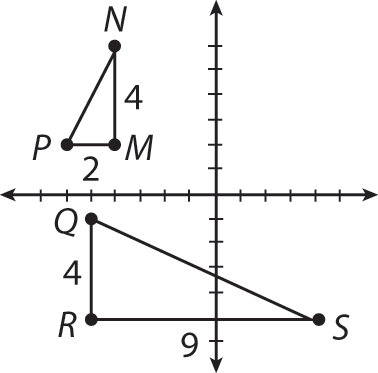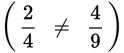# SAT Math Multiple Choice Question 967: Answer and Explanation

### Test Information

Question: 967

3. Two triangles are graphed on a coordinate plane. Triangle MNP has vertices M(–4, 2), N(–4, 6), and P(–6, 2). Triangle QRS has vertices Q(–5, –1), R(–5, –5), and S(4, –5). Which of the following statements is true?

• A. ΔMNP is congruent to ΔQRS.
• B. ΔMNP is similar to ΔQRS.
• C. ΔMNP is similar to ΔRSQ.
• D. The triangles are neither congruent nor similar.

Explanation:

D

Difficulty: Medium

Category: Additional Topics in Math / Geometry

Strategic Advice: When you're given coordinates for the vertices of two triangles and asked to compare the triangles in some way, drawing a sketch is definitely the best way to go.

Getting to the Answer: Draw a quick sketch and label any lengths that are obvious from the coordinates of the points. Because there are some repeated x- and y-coordinates, finding side lengths is likely to be easier than you might initially think.Notice that the triangles are clearly not congruent (the same size), so you can eliminate A. Both triangles are right triangles, so they could be similar. However, the ratios of corresponding pairs of sides are not equal, so the triangles are neither similar nor congruent, which is (D).